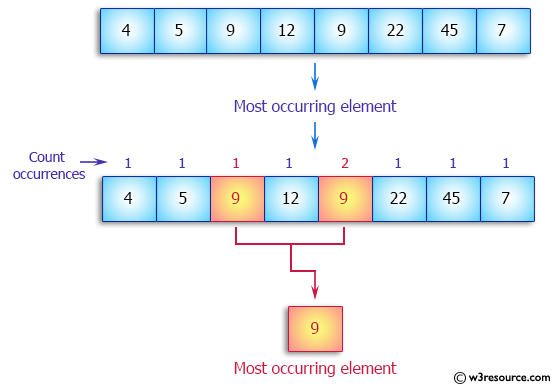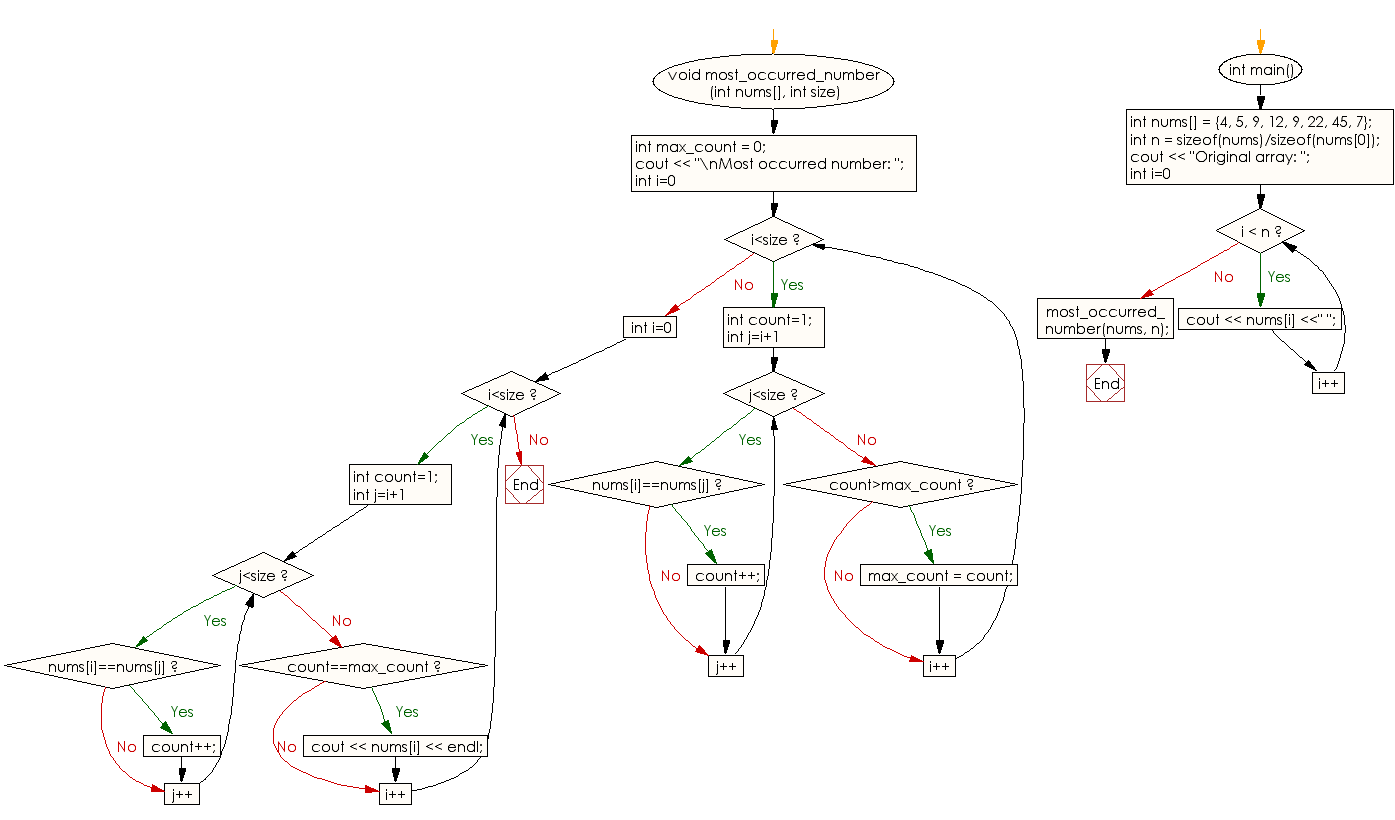﻿ C++ : Find the most occurring element in an array# C++ Exercises: Find the most occurring element in an array of integers

## C++ Array: Exercise-7 with Solution

Write a C++ program to find the most occurring element in an array of integers.

Pictorial Presentation:Sample Solution:

C++ Code :

``````#include<iostream>
using namespace std;

void most_occurred_number(int nums[], int size)
{
int max_count = 0;
cout << "\nMost occurred number: ";
for (int i=0; i<size; i++)
{
int count=1;
for (int j=i+1;j<size;j++)
if (nums[i]==nums[j])
count++;
if (count>max_count)
max_count = count;
}

for (int i=0;i<size;i++)
{
int count=1;
for (int j=i+1;j<size;j++)
if (nums[i]==nums[j])
count++;
if (count==max_count)
cout << nums[i] << endl;
}
}

int main()
{
int nums[] = {4, 5, 9, 12, 9, 22, 45, 7};
int n = sizeof(nums)/sizeof(nums);
cout << "Original array: ";
for (int i=0; i < n; i++)
cout << nums[i] <<" ";
most_occurred_number(nums, n);
}
``````

Sample Output:

```Original array: 4 5 9 12 9 22 45 7
Most occurred number: 9
```

Flowchart:C++ Code Editor:

What is the difficulty level of this exercise?

Test your Programming skills with w3resource's quiz.

﻿